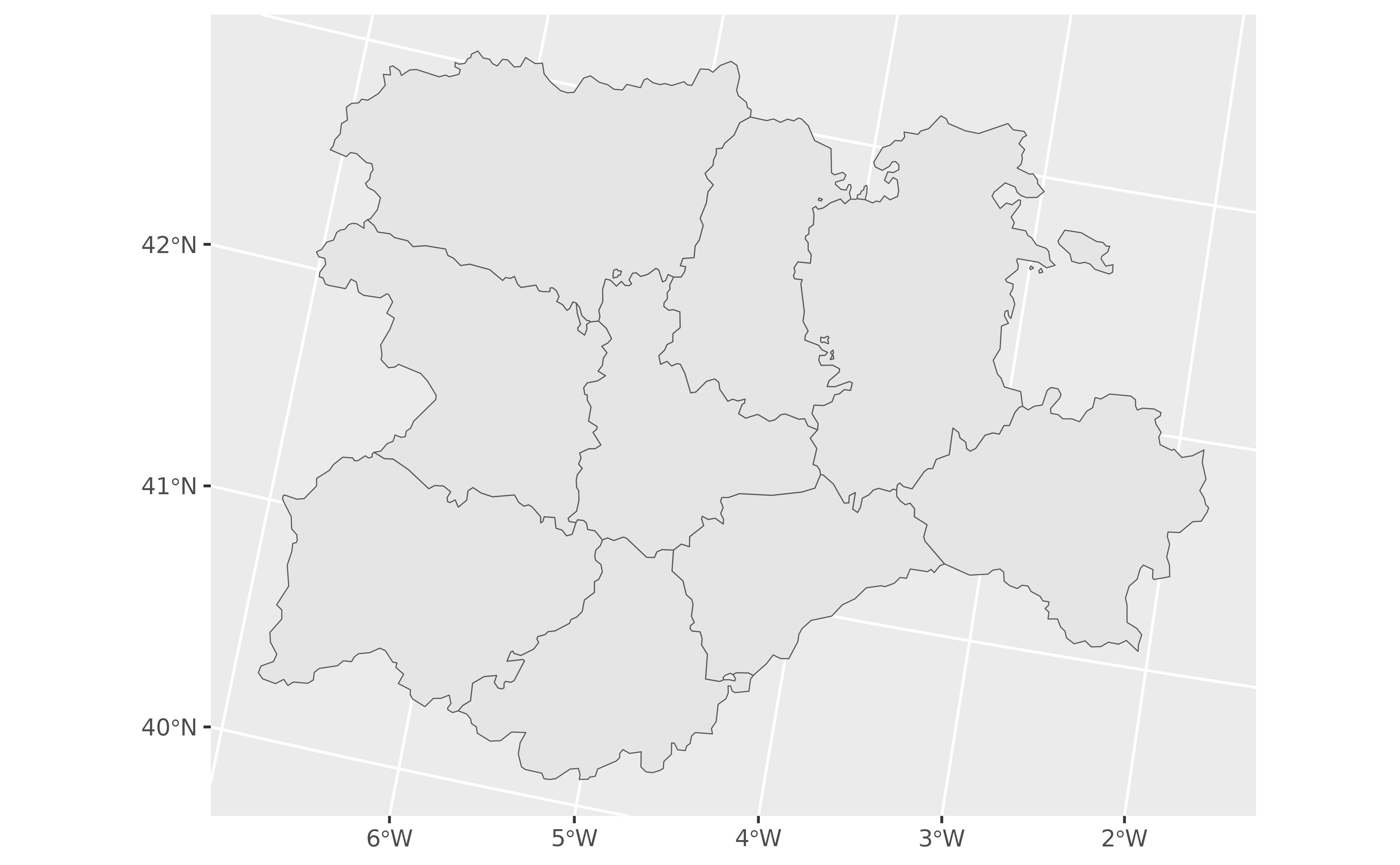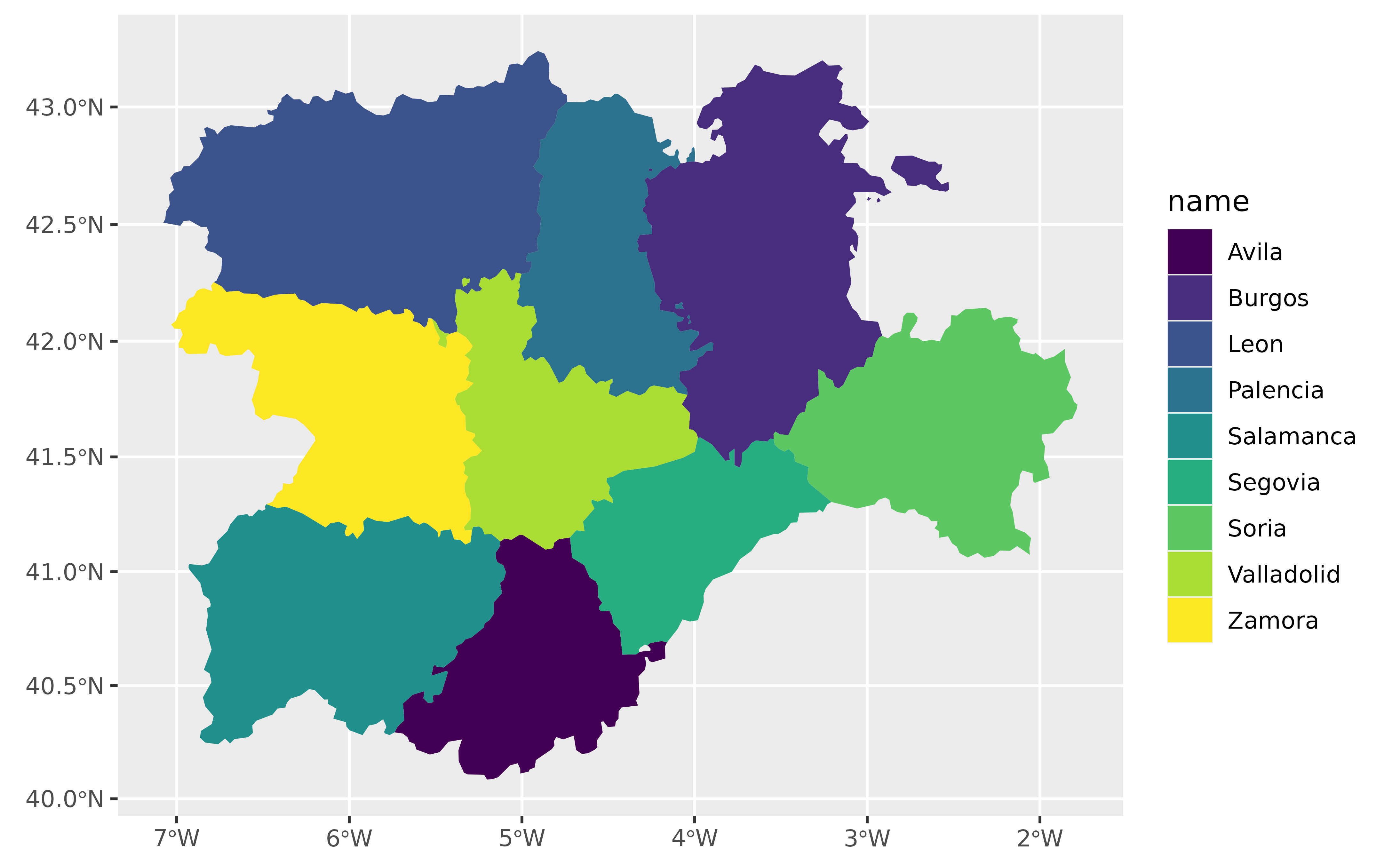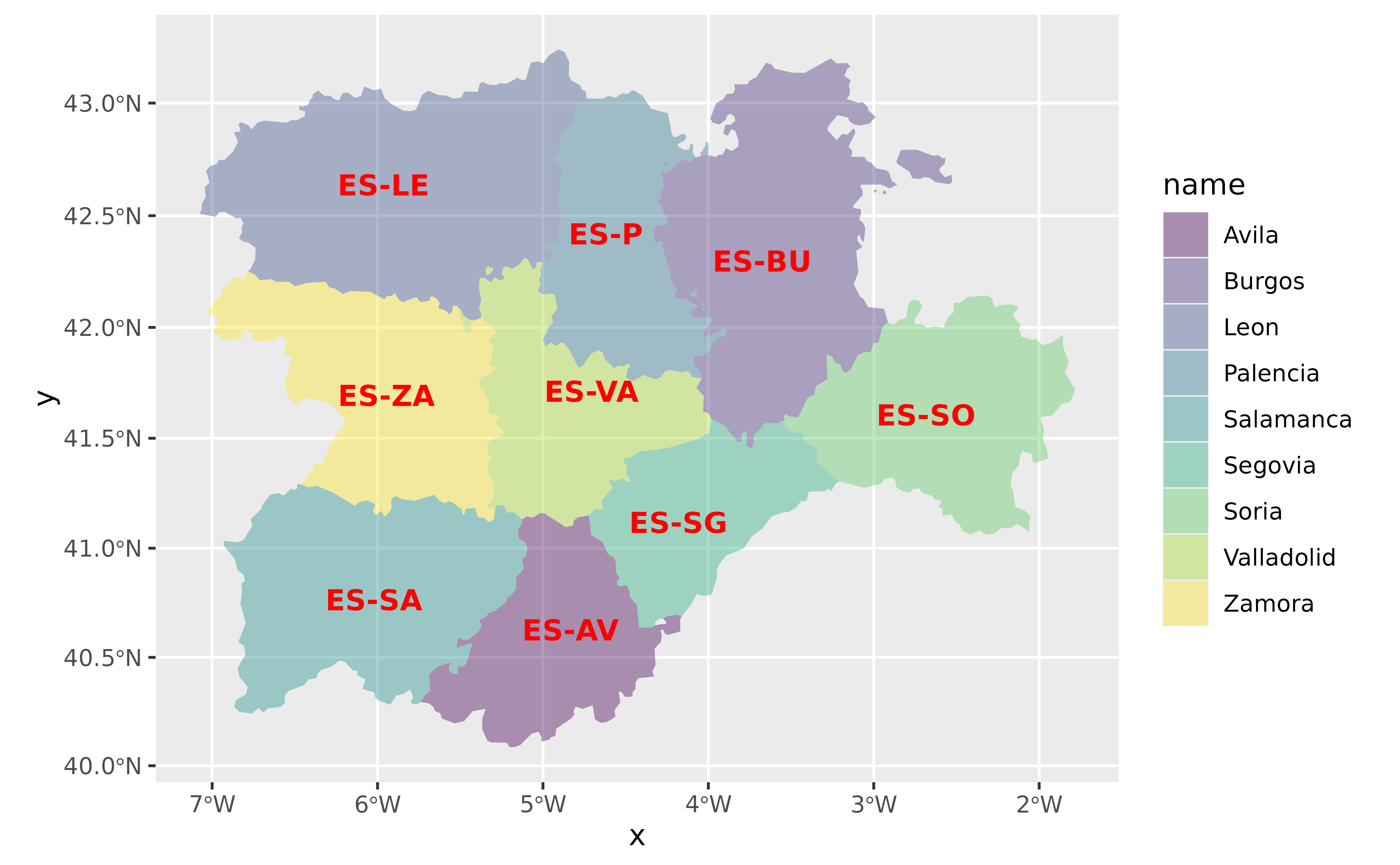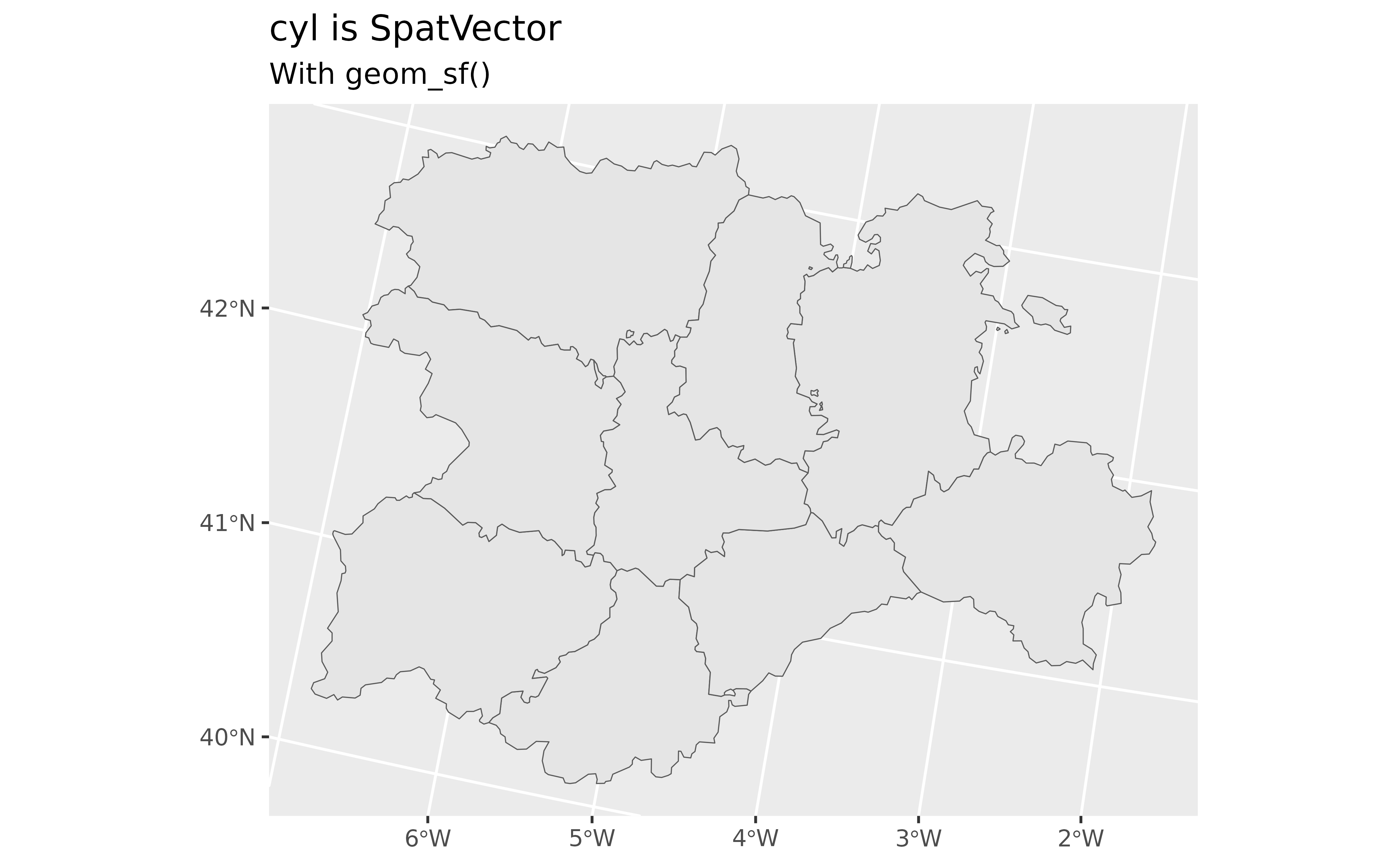Wrappers of ggplot2::geom_sf() family used to visualise SpatVector objects (see terra::vect()).

## Usage

geom_spatvector(
mapping = aes(),
data = NULL,
na.rm = FALSE,
show.legend = NA,
...
)

geom_spatvector_label(
mapping = aes(),
data = NULL,
na.rm = FALSE,
show.legend = NA,
...,
nudge_x = 0,
nudge_y = 0,
label.size = 0.25,
inherit.aes = TRUE
)

geom_spatvector_text(
mapping = aes(),
data = NULL,
na.rm = FALSE,
show.legend = NA,
...,
nudge_x = 0,
nudge_y = 0,
check_overlap = FALSE,
inherit.aes = TRUE
)

stat_spatvector(
mapping = NULL,
data = NULL,
geom = "rect",
position = "identity",
na.rm = FALSE,
show.legend = NA,
inherit.aes = TRUE,
...
)

## Arguments

mapping

Set of aesthetic mappings created by aes(). If specified and inherit.aes = TRUE (the default), it is combined with the default mapping at the top level of the plot. You must supply mapping if there is no plot mapping.

data

A SpatVector object, see terra::vect().

na.rm

If FALSE, the default, missing values are removed with a warning. If TRUE, missing values are silently removed.

show.legend

logical. Should this layer be included in the legends? NA, the default, includes if any aesthetics are mapped. FALSE never includes, and TRUE always includes.

You can also set this to one of "polygon", "line", and "point" to override the default legend.

...

Other arguments passed on to ggplot2::geom_sf() functions. These are often aesthetics, used to set an aesthetic to a fixed value, like colour = "red" or linewidth = 3.

nudge_x, nudge_y

Horizontal and vertical adjustment to nudge labels by. Useful for offsetting text from points, particularly on discrete scales. Cannot be jointly specified with position.

label.size

Size of label border, in mm.

inherit.aes

If FALSE, overrides the default aesthetics, rather than combining with them. This is most useful for helper functions that define both data and aesthetics and shouldn't inherit behaviour from the default plot specification, e.g. borders().

check_overlap

If TRUE, text that overlaps previous text in the same layer will not be plotted. check_overlap happens at draw time and in the order of the data. Therefore data should be arranged by the label column before calling geom_text(). Note that this argument is not supported by geom_label().

geom

The geometric object to use to display the data, either as a ggproto Geom subclass or as a string naming the geom stripped of the geom_ prefix (e.g. "point" rather than "geom_point")

position

Position adjustment, either as a string naming the adjustment (e.g. "jitter" to use position_jitter), or the result of a call to a position adjustment function. Use the latter if you need to change the settings of the adjustment.

A ggplot2 layer

## Details

These functions are wrappers of ggplot2::geom_sf() functions. Since a fortify.SpatVector() method is provided, ggplot2 treat a SpatVector in the same way that a sf object. A side effect is that you can use ggplot2::geom_sf() directly with SpatVectors.

See ggplot2::geom_sf() for details on aesthetics, etc.

## terra equivalent

terra::plot()

ggplot2::geom_sf()

Other ggplot2 utils: autoplot.Spat, fortify.Spat, geom_spat_contour, geom_spatraster_rgb(), geom_spatraster(), stat_spat_coordinates()

## Examples

# \donttest{
# Create a SpatVector
extfile <- system.file("extdata/cyl.gpkg", package = "tidyterra")

cyl <- terra::vect(extfile)
class(cyl)
#>  "SpatVector"
#> attr(,"package")
#>  "terra"

library(ggplot2)

ggplot(cyl) +
geom_spatvector()# With params

ggplot(cyl) +
geom_spatvector(aes(fill = name), color = NA) +
scale_fill_viridis_d() +
coord_sf(crs = 3857)ggplot(cyl) +
geom_spatvector(aes(fill = name), color = NA) +
geom_spatvector_text(aes(label = iso2),
fontface = "bold",
color = "red"
) +
scale_fill_viridis_d(alpha = 0.4) +
coord_sf(crs = 3857)# You can use now geom_sf with SpatVectors!

ggplot(cyl) +
geom_sf() +
labs(
title = paste("cyl is", as.character(class(cyl))),
subtitle = "With geom_sf()"
)# }